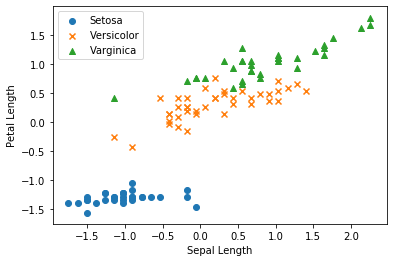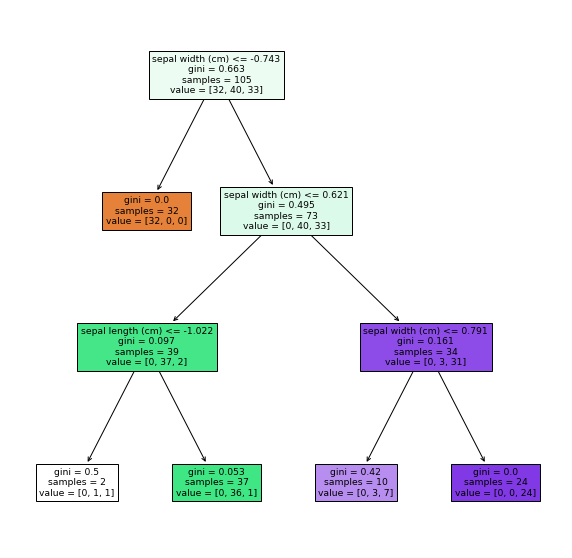# [PYTHON] Decision tree (for beginners) -Code edition-

This time, we will summarize the implementation of decision trees (classifications).

## ■ Procedure of decision tree

Proceed with the next 7 steps.

1. Preparation of module
2. Data preparation
3. Data visualization
4. Creating a model
5. Model plot
6. Predict classification
7. Model evaluation

## 1. Preparation of module

First, import the required modules.

``````
import numpy as np
import pandas as pd
import matplotlib.pyplot as plt

#Module for standardization (distributed normalization)
from sklearn.preprocessing import StandardScaler

#Module that separates training data and test data
from sklearn.model_selection import train_test_split

#Module to execute decision tree
from sklearn.tree import DecisionTreeClassifier

#Module to plot decision tree
from sklearn.tree import plot_tree
``````

## 2. Data preparation

This time, we will use the iris dataset to classify three types.

Get the data first, standardize it, and then split it.

``````

#Divide into objective variable and explanatory variable (feature amount)
X, y = iris.data[:, [0, 2]], iris.target

#Standardization (distributed normalization)
std = StandardScaler()
X = std.fit_transform(X)

#Divide into training data and test data
X_train, X_test, y_train, y_test = train_test_split(X, y, test_size = 0.3, random_state = 123)
``````

To make it easier to plot, we have narrowed down the features to two. (Sepal Length / Petal Lengh only)

In standardization, for example, when there are 2-digit and 4-digit features (explanatory variables), the influence of the latter becomes large. The scale is aligned by setting the average to 0 and the variance to 1 for all features.

In random_state, the seed value is fixed and the data division result is set to be the same every time.

## 3. Data visualization

Let's plot the data before classifying by SVM.

``````
#Creating drawing objects and subplots
fig, ax = plt.subplots()

#Setosa plot
ax.scatter(X_train[y_train == 0, 0], X_train[y_train == 0, 1],
marker = 'o', label = 'Setosa')

#Versicolor plot
ax.scatter(X_train[y_train == 1, 0], X_train[y_train == 1, 1],
marker = 'x', label = 'Versicolor')

#Varginica plot
ax.scatter(X_train[y_train == 2, 0], X_train[y_train == 2, 1],
marker = 'x', label = 'Varginica')

#Axis label settings
ax.set_xlabel('Sepal Length')
ax.set_ylabel('Petal Length')

#Legend settings
ax.legend(loc = 'best')

plt.show()
``````

Plot with features corresponding to Setosa (y_train == 0) (0: Sepal Lengh on the horizontal axis, 1: Petal Length on the vertical axis) Plot with features corresponding to Versicolor (y_train == 1) (0: Sepal Lengh on the horizontal axis, 1: Petal Length on the vertical axis) Plot with features corresponding to Varginica (y_train == 2) (0: Sepal Lengh on the horizontal axis, 1: Petal Length on the vertical axis)

Output result## 4. Creating a model

First, create an execution function (instance) of the decision tree and apply it to the training data.

``````
#Create an instance
tree = DecisionTreeClassifier(max_depth = 3)

#Create a model from training data
tree.fit(X_train, y_train)
``````

max_depth (tree depth) is a hyperparameter You can adjust it yourself while looking at the output values and plots.

## 5. Model plot

Since we were able to create a model of the decision tree from the training data Plot and check how the classification is done.

``````
#Set the size of the plot
fig, ax = plt.subplots(figsize=(10, 10))

# plot_Use the tree method (argument: instance of decision tree, list of features)
plot_tree(tree, feature_names=iris.feature_names, filled=True)

plt.show()
``````

In many cases, it is plotted with GraphViz, but since it needs to be installed and passed through the path, This time, we will draw with the plot_tree method.

Output result## 6. Predict classification

Now that the model is complete, let's predict the classification.

``````#Predict classification results
y_pred = tree.predict(X_test)

#Output predicted value and correct answer value
print(y_pred)
print(y_test)
``````

Output result

``````
y_pred: [2 2 2 1 0 1 1 0 0 1 2 0 1 2 2 2 0 0 1 0 0 2 0 2 0 0 0 2 2 0 2 2 0 0 1 1 2
0 0 1 1 0 2 2 2]
y_test: [1 2 2 1 0 2 1 0 0 1 2 0 1 2 2 2 0 0 1 0 0 2 0 2 0 0 0 2 2 0 2 2 0 0 1 1 2
0 0 1 1 0 2 2 2]
``````

0：Setosa　1：Versicolor　2：Verginica

## 7. Model evaluation

Since there are three types of classification this time, we will evaluate by the correct answer rate.

``````
print(tree.score(X_test, y_test))
``````

Output result

``````
Accuracy: 0.9555555555555556
``````

From the above, we were able to evaluate the classification in Setosa, Versicolor, and Verginica.

## ■ Finally

In the decision tree, we will create and evaluate the model based on the steps 1 to 7 above.

This time, for beginners, I have summarized only the implementation (code). Looking at the timing in the future, I would like to write an article about theory (mathematical formula).